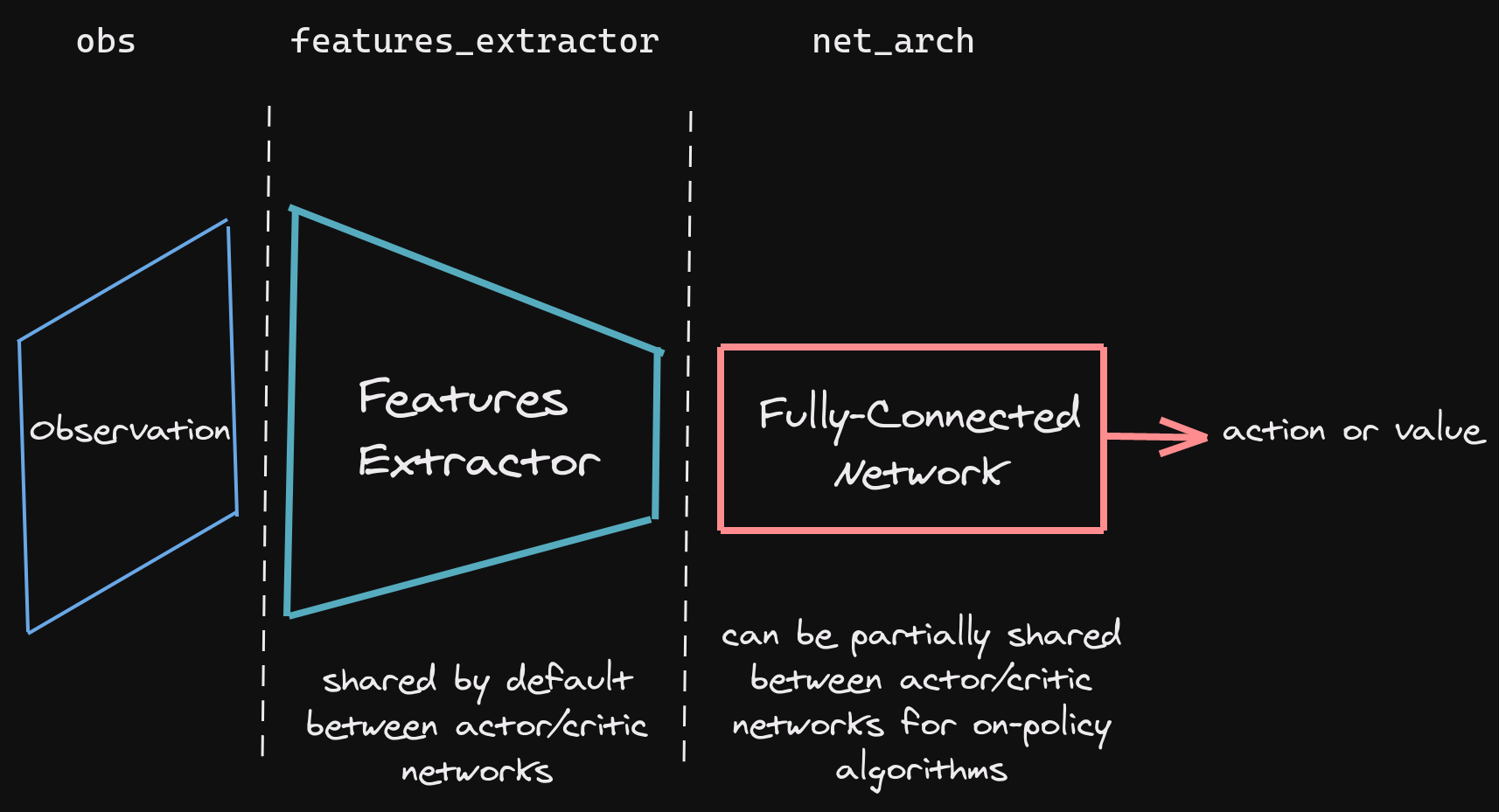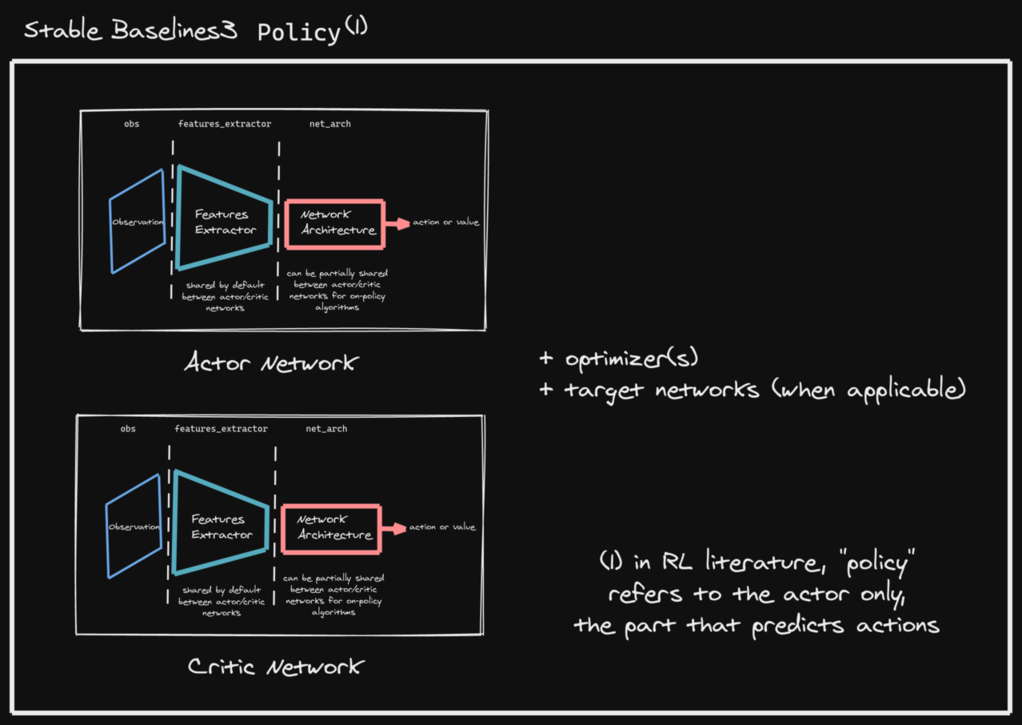# Policy Networks

Stable Baselines3 provides policy networks for images (CnnPolicies), other type of input features (MlpPolicies) and multiple different inputs (MultiInputPolicies).

Warning

For A2C and PPO, continuous actions are clipped during training and testing (to avoid out of bound error). SAC, DDPG and TD3 squash the action, using a `tanh()` transformation, which handles bounds more correctly.

## SB3 Policy

SB3 networks are separated into two mains parts (see figure below):

• A features extractor (usually shared between actor and critic when applicable, to save computation) whose role is to extract features (i.e. convert to a feature vector) from high-dimensional observations, for instance, a CNN that extracts features from images. This is the `features_extractor_class` parameter. You can change the default parameters of that features extractor by passing a `features_extractor_kwargs` parameter.

• A (fully-connected) network that maps the features to actions/value. Its architecture is controlled by the `net_arch` parameter.

Note

All observations are first pre-processed (e.g. images are normalized, discrete obs are converted to one-hot vectors, …) before being fed to the features extractor. In the case of vector observations, the features extractor is just a `Flatten` layer.SB3 policies are usually composed of several networks (actor/critic networks + target networks when applicable) together with the associated optimizers.

Each of these network have a features extractor followed by a fully-connected network.

Note

When we refer to “policy” in Stable-Baselines3, this is usually an abuse of language compared to RL terminology. In SB3, “policy” refers to the class that handles all the networks useful for training, so not only the network used to predict actions (the “learned controller”).## Default Network Architecture

The default network architecture used by SB3 depends on the algorithm and the observation space. You can visualize the architecture by printing `model.policy` (see issue #329).

For 1D observation space, a 2 layers fully connected net is used with:

• 64 units (per layer) for PPO/A2C/DQN

• 256 units for SAC

• [400, 300] units for TD3/DDPG (values are taken from the original TD3 paper)

For image observation spaces, the “Nature CNN” (see code for more details) is used for feature extraction, and SAC/TD3 also keeps the same fully connected network after it. The other algorithms only have a linear layer after the CNN. The CNN is shared between actor and critic for A2C/PPO (on-policy algorithms) to reduce computation. Off-policy algorithms (TD3, DDPG, SAC, …) have separate feature extractors: one for the actor and one for the critic, since the best performance is obtained with this configuration.

For mixed observations (dictionary observations), the two architectures from above are used, i.e., CNN for images and then two layers fully-connected network (with a smaller output size for the CNN).

## Custom Network Architecture

One way of customising the policy network architecture is to pass arguments when creating the model, using `policy_kwargs` parameter:

Note

An extra linear layer will be added on top of the layers specified in `net_arch`, in order to have the right output dimensions and activation functions (e.g. Softmax for discrete actions).

In the following example, as CartPole’s action space has a dimension of 2, the final dimensions of the `net_arch`’s layers will be:

```        obs
<4>
/            \
<32>          <32>
|              |
<32>          <32>
|              |
<2>            <1>
action         value
```
```import gymnasium as gym
import torch as th

from stable_baselines3 import PPO

# Custom actor (pi) and value function (vf) networks
# of two layers of size 32 each with Relu activation function
# Note: an extra linear layer will be added on top of the pi and the vf nets, respectively
policy_kwargs = dict(activation_fn=th.nn.ReLU,
net_arch=dict(pi=[32, 32], vf=[32, 32]))
# Create the agent
model = PPO("MlpPolicy", "CartPole-v1", policy_kwargs=policy_kwargs, verbose=1)
# Retrieve the environment
env = model.get_env()
# Train the agent
model.learn(total_timesteps=20_000)
# Save the agent
model.save("ppo_cartpole")

del model
# the policy_kwargs are automatically loaded
```

## Custom Feature Extractor

If you want to have a custom features extractor (e.g. custom CNN when using images), you can define class that derives from `BaseFeaturesExtractor` and then pass it to the model when training.

Note

For on-policy algorithms, the features extractor is shared by default between the actor and the critic to save computation (when applicable). However, this can be changed setting `share_features_extractor=False` in the `policy_kwargs` (both for on-policy and off-policy algorithms).

```import torch as th
import torch.nn as nn
from gymnasium import spaces

from stable_baselines3 import PPO
from stable_baselines3.common.torch_layers import BaseFeaturesExtractor

class CustomCNN(BaseFeaturesExtractor):
"""
:param observation_space: (gym.Space)
:param features_dim: (int) Number of features extracted.
This corresponds to the number of unit for the last layer.
"""

def __init__(self, observation_space: spaces.Box, features_dim: int = 256):
super().__init__(observation_space, features_dim)
# We assume CxHxW images (channels first)
# Re-ordering will be done by pre-preprocessing or wrapper
n_input_channels = observation_space.shape
self.cnn = nn.Sequential(
nn.ReLU(),
nn.ReLU(),
nn.Flatten(),
)

# Compute shape by doing one forward pass
n_flatten = self.cnn(
th.as_tensor(observation_space.sample()[None]).float()
).shape

self.linear = nn.Sequential(nn.Linear(n_flatten, features_dim), nn.ReLU())

def forward(self, observations: th.Tensor) -> th.Tensor:
return self.linear(self.cnn(observations))

policy_kwargs = dict(
features_extractor_class=CustomCNN,
features_extractor_kwargs=dict(features_dim=128),
)
model = PPO("CnnPolicy", "BreakoutNoFrameskip-v4", policy_kwargs=policy_kwargs, verbose=1)
model.learn(1000)
```

## Multiple Inputs and Dictionary Observations

Stable Baselines3 supports handling of multiple inputs by using `Dict` Gym space. This can be done using `MultiInputPolicy`, which by default uses the `CombinedExtractor` features extractor to turn multiple inputs into a single vector, handled by the `net_arch` network.

By default, `CombinedExtractor` processes multiple inputs as follows:

1. If input is an image (automatically detected, see `common.preprocessing.is_image_space`), process image with Nature Atari CNN network and output a latent vector of size `256`.

2. If input is not an image, flatten it (no layers).

3. Concatenate all previous vectors into one long vector and pass it to policy.

Much like above, you can define custom features extractors. The following example assumes the environment has two keys in the observation space dictionary: “image” is a (1,H,W) image (channel first), and “vector” is a (D,) dimensional vector. We process “image” with a simple downsampling and “vector” with a single linear layer.

```import gymnasium as gym
import torch as th
from torch import nn

from stable_baselines3.common.torch_layers import BaseFeaturesExtractor

class CustomCombinedExtractor(BaseFeaturesExtractor):
def __init__(self, observation_space: gym.spaces.Dict):
# We do not know features-dim here before going over all the items,
# so put something dummy for now. PyTorch requires calling
super().__init__(observation_space, features_dim=1)

extractors = {}

total_concat_size = 0
# We need to know size of the output of this extractor,
# so go over all the spaces and compute output feature sizes
for key, subspace in observation_space.spaces.items():
if key == "image":
# We will just downsample one channel of the image by 4x4 and flatten.
# Assume the image is single-channel (subspace.shape == 0)
extractors[key] = nn.Sequential(nn.MaxPool2d(4), nn.Flatten())
total_concat_size += subspace.shape // 4 * subspace.shape // 4
elif key == "vector":
# Run through a simple MLP
extractors[key] = nn.Linear(subspace.shape, 16)
total_concat_size += 16

self.extractors = nn.ModuleDict(extractors)

# Update the features dim manually
self._features_dim = total_concat_size

def forward(self, observations) -> th.Tensor:
encoded_tensor_list = []

# self.extractors contain nn.Modules that do all the processing.
for key, extractor in self.extractors.items():
encoded_tensor_list.append(extractor(observations[key]))
# Return a (B, self._features_dim) PyTorch tensor, where B is batch dimension.
return th.cat(encoded_tensor_list, dim=1)
```

## On-Policy Algorithms

### Custom Networks

If you need a network architecture that is different for the actor and the critic when using `PPO`, `A2C` or `TRPO`, you can pass a dictionary of the following structure: `dict(pi=[<actor network architecture>], vf=[<critic network architecture>])`.

For example, if you want a different architecture for the actor (aka `pi`) and the critic ( value-function aka `vf`) networks, then you can specify `net_arch=dict(pi=[32, 32], vf=[64, 64])`.

Otherwise, to have actor and critic that share the same network architecture, you only need to specify `net_arch=[128, 128]` (here, two hidden layers of 128 units each, this is equivalent to `net_arch=dict(pi=[128, 128], vf=[128, 128])`).

If shared layers are needed, you need to implement a custom policy network (see advanced example below).

#### Examples

Same architecture for actor and critic with two layers of size 128: `net_arch=[128, 128]`

```        obs
/            \
<128>          <128>
|              |
<128>          <128>
|              |
action         value
```

Different architectures for actor and critic: `net_arch=dict(pi=[32, 32], vf=[64, 64])`

```        obs
/            \
<32>          <64>
|              |
<32>          <64>
|              |
action         value
```

If your task requires even more granular control over the policy/value architecture, you can redefine the policy directly:

```from typing import Callable, Dict, List, Optional, Tuple, Type, Union

from gymnasium import spaces
import torch as th
from torch import nn

from stable_baselines3 import PPO
from stable_baselines3.common.policies import ActorCriticPolicy

class CustomNetwork(nn.Module):
"""
Custom network for policy and value function.
It receives as input the features extracted by the features extractor.

:param feature_dim: dimension of the features extracted with the features_extractor (e.g. features from a CNN)
:param last_layer_dim_pi: (int) number of units for the last layer of the policy network
:param last_layer_dim_vf: (int) number of units for the last layer of the value network
"""

def __init__(
self,
feature_dim: int,
last_layer_dim_pi: int = 64,
last_layer_dim_vf: int = 64,
):
super().__init__()

# IMPORTANT:
# Save output dimensions, used to create the distributions
self.latent_dim_pi = last_layer_dim_pi
self.latent_dim_vf = last_layer_dim_vf

# Policy network
self.policy_net = nn.Sequential(
nn.Linear(feature_dim, last_layer_dim_pi), nn.ReLU()
)
# Value network
self.value_net = nn.Sequential(
nn.Linear(feature_dim, last_layer_dim_vf), nn.ReLU()
)

def forward(self, features: th.Tensor) -> Tuple[th.Tensor, th.Tensor]:
"""
:return: (th.Tensor, th.Tensor) latent_policy, latent_value of the specified network.
If all layers are shared, then ``latent_policy == latent_value``
"""
return self.forward_actor(features), self.forward_critic(features)

def forward_actor(self, features: th.Tensor) -> th.Tensor:
return self.policy_net(features)

def forward_critic(self, features: th.Tensor) -> th.Tensor:
return self.value_net(features)

class CustomActorCriticPolicy(ActorCriticPolicy):
def __init__(
self,
observation_space: spaces.Space,
action_space: spaces.Space,
lr_schedule: Callable[[float], float],
*args,
**kwargs,
):
# Disable orthogonal initialization
kwargs["ortho_init"] = False
super().__init__(
observation_space,
action_space,
lr_schedule,
# Pass remaining arguments to base class
*args,
**kwargs,
)

def _build_mlp_extractor(self) -> None:
self.mlp_extractor = CustomNetwork(self.features_dim)

model = PPO(CustomActorCriticPolicy, "CartPole-v1", verbose=1)
model.learn(5000)
```

## Off-Policy Algorithms

If you need a network architecture that is different for the actor and the critic when using `SAC`, `DDPG`, `TQC` or `TD3`, you can pass a dictionary of the following structure: `dict(pi=[<actor network architecture>], qf=[<critic network architecture>])`.

For example, if you want a different architecture for the actor (aka `pi`) and the critic (Q-function aka `qf`) networks, then you can specify `net_arch=dict(pi=[64, 64], qf=[400, 300])`.

Otherwise, to have actor and critic that share the same network architecture, you only need to specify `net_arch=[256, 256]` (here, two hidden layers of 256 units each).

Note

For advanced customization of off-policy algorithms policies, please take a look at the code. A good understanding of the algorithm used is required, see discussion in issue #425

```from stable_baselines3 import SAC

# Custom actor architecture with two layers of 64 units each
# Custom critic architecture with two layers of 400 and 300 units
policy_kwargs = dict(net_arch=dict(pi=[64, 64], qf=[400, 300]))
# Create the agent
model = SAC("MlpPolicy", "Pendulum-v1", policy_kwargs=policy_kwargs, verbose=1)
model.learn(5000)
```